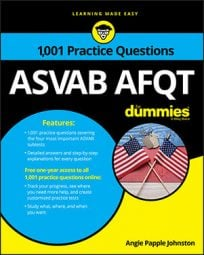##### ASVAB AFQTOn the Arithmetic Reasoning subtest on the ASVAB, two-dimensional geometry questions are usually pretty straightforward. However, if they’re presented as word problems, it’s important that you read them carefully and maybe even draw a diagram to help you visualize the problem.

## Practice questions

1. A rectangular picture frame has an interior perimeter of 38 inches. One interior side of the frame is 11 inches long. How long is the other interior side of the picture frame?

A. 12 in. B. 16 in. C. 27 in. D. 8 in.

2. A square garden with an area of 169 square meters needs to be surrounded by a walkway that’s 2 meters across. What is the walkway’s area?

A. 56 m2 B. 110 m2 C. 120 m2 D. 128 m2

1. The correct answer is Choice (D).

The formula to find the perimeter of a rectangle is P = 2l + 2w, where P represents the perimeter, l represents the length, and w represents the width.

Find the lengths by using the perimeter formula:

The other interior side of the picture frame is 8 inches long.

2. The correct answer is Choice (C).

The garden is square, so find its dimensions by finding the square root of its area:

Each side of the garden is 13 meters long.

The walkway needs to measure 2 meters across, so add 2 meters to each side to get the size of the garden and walkway together; each side is 17 meters long.

You can find the area of the entire project — the garden plus the walkway — by squaring 17: 172 = 289.

Now subtract the smaller area (the garden) from the area covering the walkway and the garden: 289 – 169 = 120. The walkway’s area is 120 square meters.